# Several ways of plotting subgraphs with matplotlib

Keywords: IPython Javascript

This paper mainly uses matplotlib to draw multiple graphs.

```%matplotlib notebook
import matplotlib
from matplotlib import pyplot as plt
plt.ion()
%matplotlib tk
from matplotlib import rcdefaults
rcdefaults()
import numpy as np
import pandas as pd
```

# Method 1: use of plot.subplot (x, x, x) and fig.add_

```X = np.linspace(-3,3, 200)
C = np.sin(X)
S = np.cos(X)

#Subplots
#       With subplot you can arrange plots in a regular grid. You need to specify the number of rows and columns and the number of the plot.
#       Note that the gridspec command is a more powerful alternative.
'''plt.figure()
plt.subplot(2, 1, 1)
plt.subplot(2, 1, 2)
plt.show()'''
plt.figure()
plt.subplot(1, 2, 1)
plt.plot(X, C)
plt.title('1st figure')
plt.subplot(1, 2, 2)
plt.plot(X, S)
plt.title('2nd figure')
plt.show()
```
```<IPython.core.display.Javascript object>
```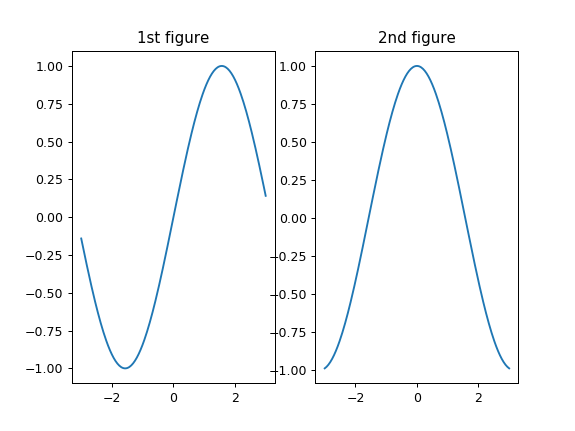```fig = plt.figure()

X = np.linspace(-3,3, 200)
C = np.sin(X)
S = np.cos(X)

ax1.plot(X,C)
ax2.plot(X,S)
plt.show()
```
```<IPython.core.display.Javascript object>
```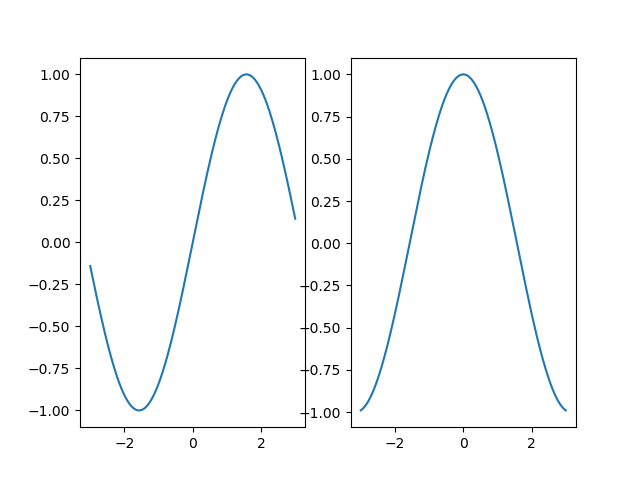# Method 2: plt.subplot2grid

Suitable for irregular subgraph Division

```# Define figure
plt.figure()
# figure is divided into three rows and three columns to obtain the handle of the first subgraph. The span of the first subgraph is 1 row and three columns, and the starting point is table (0, 0)
ax1 = plt.subplot2grid((3, 3), (0, 0), colspan = 3, rowspan = 1)
ax1.plot([0, 1], [0, 1])
ax1.set_title('Test')

# figure is divided into three rows and three columns to obtain the handle of the second subgraph. The span of the second subgraph is one row and three columns, and the starting point is table (1, 0)
ax2 = plt.subplot2grid((3, 3), (1, 0), colspan = 2, rowspan = 1)
ax2.plot([0, 1], [0, 1])

# figure is divided into three rows and three columns to obtain the handle of the third subgraph. The span of the third subgraph is one row and one column, and the starting point is table (1, 2)
ax3 = plt.subplot2grid((3, 3), (1, 2), colspan = 1, rowspan = 1)
ax3.plot([0, 1], [0, 1])

# figure is divided into three rows and three columns to obtain the handle of the fourth subgraph. The span of the fourth subgraph is one row and three columns, and the starting point is table (2, 0)
ax4 = plt.subplot2grid((3, 3), (2, 0), colspan = 3, rowspan = 1)
ax4.plot([0, 1], [0, 1])

plt.show()
```
```<IPython.core.display.Javascript object>
```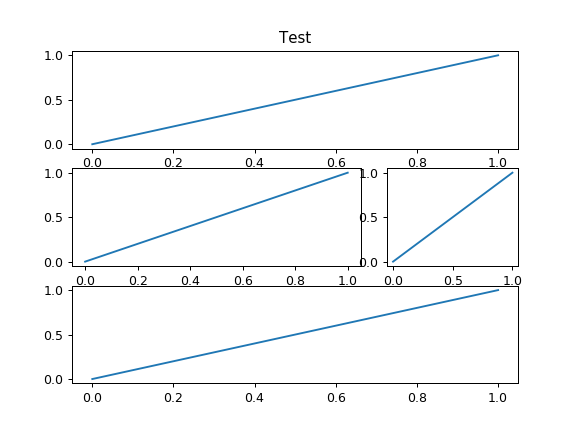# Method 3: matplotlib.gridspec.GridSpec

It is also applicable to irregular graph Division

```
# Define figure
plt.figure()
# Separate figure
gs = matplotlib.gridspec.GridSpec(3, 3)
ax1 = plt.subplot(gs[0, :])
ax2 = plt.subplot(gs[1, 0:2])
ax3 = plt.subplot(gs[1, 2])
ax4 = plt.subplot(gs[2, :])

# Drawing images
ax1.plot([0, 1], [0, 1])
ax1.set_title('Test')

ax2.plot([0, 1], [0, 1])

ax3.plot([0, 1], [0, 1])

ax4.plot([0, 1], [0, 1])

plt.show()
```
```<IPython.core.display.Javascript object>
```# Method 4: plt.subplots

```# Dividing figure
fig, ((ax11, ax12), (ax21, ax22)) = plt.subplots(2, 2, sharex = True, sharey = True,figsize=(10.0, 6.0))
# Drawing images
ax11.scatter([0, 0.5], [0, 1])
ax12.scatter([0, 1], [0, 1])
ax21.scatter([0, 1], [0, -1])
ax22.scatter([0, -1], [0, 1])

plt.show()
```
```<IPython.core.display.Javascript object>
```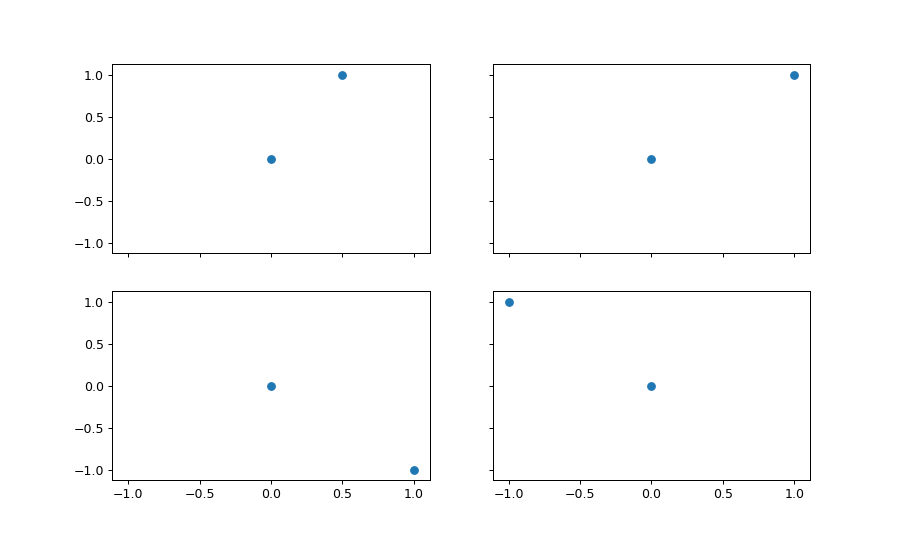```# Dividing figure
# Do not use this method. If there is a problem in drawing the image, it will not be displayed
fig, ((ax11, ax12), (ax21, ax22)) = plt.subplots(2, 2, sharex = True, sharey = True )
# Drawing images
ax11.scatter([0, 0.5], [0, 1])
ax12.scatter([0, 1], [0, 1])
ax21.scatter([0, 1], [0, -1])
ax22.scatter([0, -1], [0, 1])
plt.tight_layout()
plt.show()
```
```<IPython.core.display.Javascript object>
```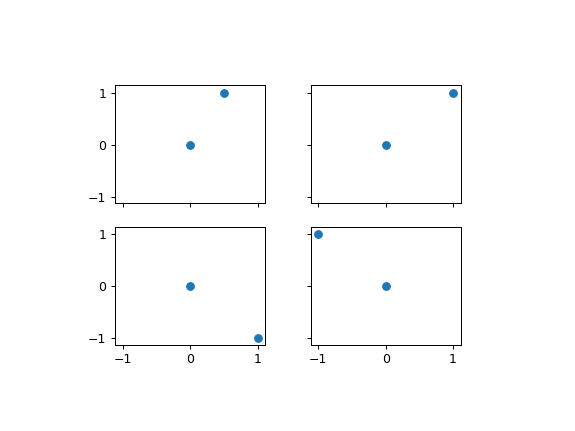# Subgraph Title settings

Sub image unified title setting.
In fact, the idea is to create the whole sub image, and then set the scale, annotation and other parts of the image to display only the title of the image.

```fig, big_axes = plt.subplots(figsize=(9, 9) , nrows=3, ncols=1, sharey=True)

for row, big_ax in enumerate(big_axes, start=1):
big_ax.set_title("Subplot row %s \n" % row, fontsize=16)

# Turn off axis lines and ticks of the big subplot
# obs alpha is 0 in RGBA string!
big_ax.tick_params(labelcolor=(0,0,0,0), top='off', bottom='off', left='off', right='off')
# removes the white frame
big_ax._frameon = False

for i in range(1,10):
ax.set_title('Plot title ' + str(i))

fig.set_facecolor('w')
plt.tight_layout()
plt.show()
```
```<IPython.core.display.Javascript object>
```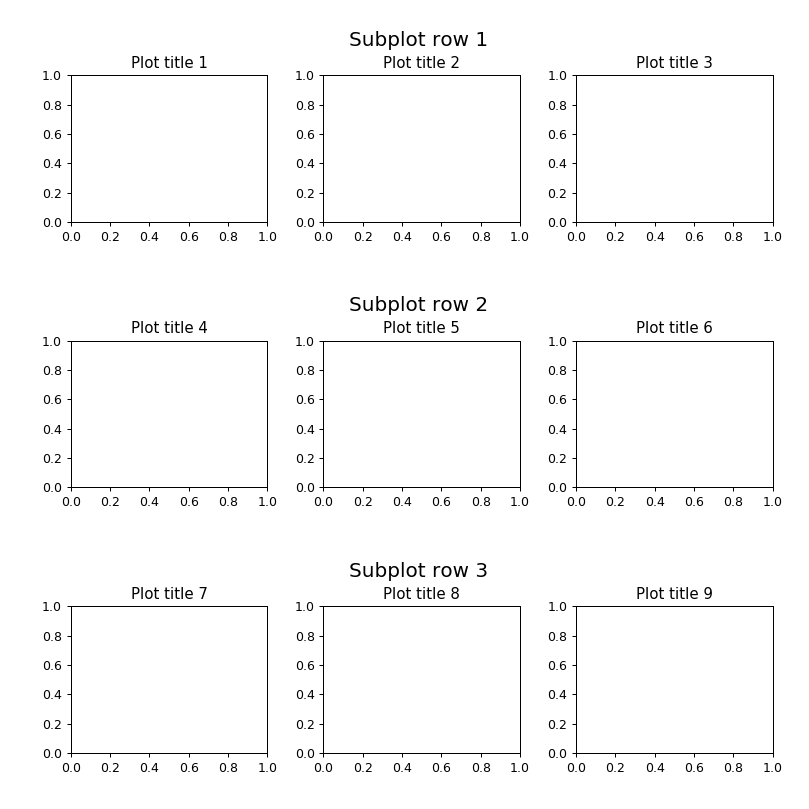# Subgraph spacing control

• The adjustment of the external edge of the image can be automatically controlled by using PLT. Tight'layout(). This method can not control the interval between images very well.

• If you want to control both the outside edge of the image and the blank area between images, use the command: